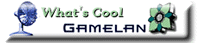### Spirograph

The Spirograph applet is written using Java. You must turn on Java or have a Java-enabled browser such as Firefox or Microsoft IE to see this applet.

You can display this spirograph on your own web page. See below.

What is a Spirograph?

A Spirograph is a curve formed by rolling a circle inside or outside of another circle. The pen is placed at any point on the rolling circle. If the radius of fixed circle is R, the radius of moving circle is r, and the offset of the pen point in the moving circle is O, then the equations of the resulting curve is defined by:

x = (R+r)*cos(t) - O*cos(((R+r)/r)*t)
y = (R+r)*sin(t) - O*sin(((R+r)/r)*t)
(moving circle outside the fixed circle)

x = (R-r)*cos(t) + O*cos(((R-r)/r)*t)
y = (R-r)*sin(t) - O*sin(((R-r)/r)*t)
(moving circle inside the fixed circle)

How to use it?

Here is how you can use the controls in this Spirograph applet:

• The first three scroll bars in the control panel let you change R, r and O respectively.

• You can use the next three scroll bars to change the color of the drawing. These scroll bars change the red, green and blue values of the color (in the range 0-255) respectively.

• The last scroll bar lets you choose the number of iterations for the Spirograph. Move it to beginning and then slowly increase it to see how many iterations it takes to complete the spirograph.

• You can use the Random button to select random values for the radii and color.

Embed following HTML code in your web page. That's all! Feel free to change the initial values of the radii in the code below.

```<applet codebase="https://wordsmith.org/anu/java/" archive="Spiro.jar" code="Spiro.class" width=680 height=400>
<param name="R" VALUE="81">
<param name="smallr" VALUE="2">
<param name="O" VALUE="71">
<param name="movingCircleOut" VALUE="false">
<param name="redBits" VALUE="242">
<param name="greenBits" VALUE="255">
<param name="blueBits" VALUE="139">
<param name="colorMode" VALUE="multi">
</applet>
<br>
Created by <A HREF="https://wordsmith.org/anu/">Anu </A>
<a href="https://wordsmith.org/anu/">Garg</A>.
<p>
```

I have suggestions for improving this applet?

Your comments are welcome at garg AT wordsmith.org. (replace AT with @)

Compressed zip file with source, bytecode and all supporting files: spirograph.zip

Where can I buy the good old spirograph I can touch and hold?

If you'd rather have a spirograph made of atoms rather than bits, you can buy one at Amazon.com: try this or this.

AwardsAnu Garg